Select Page

# 12 Science CBSE Maths Answers for MCQ Continuity and Differentiability in English

12 Science CBSE Maths Answers for MCQ Continuity and Differentiability in English to enable students to get Answers in a narrative video format for the specific question.

Expert Teacher provides 12 Science CBSE Maths Answers for MCQ Continuity and Differentiability through Video Answers in English language. This video solution will be useful for students to understand how to write an answer in exam in order to score more marks. This teacher uses a narrative style for a question from Continuity and Differentiability not only to explain the proper method of answering question, but deriving right answer too.

Please find the question below and view the Answer in a narrative video format.

Question:

## Similar Questions from CBSE, 12th Science, Maths, Continuity and Differentiability

Question 1 : Using the fact that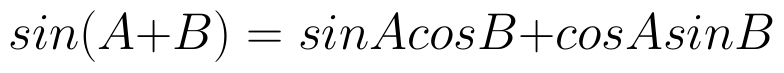and the differentiation, obtain the sum formula for cosines. (View Answer Video)

Question 2 : Differentiate the function w.r.t.x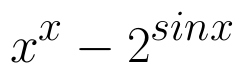. (View Answer Video)

Question 3 : If x and y are connected parametrically by the equation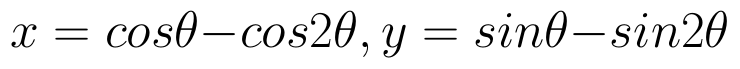, without eliminating the parameter, find. (View Answer Video)

Question 4 : Findfor the function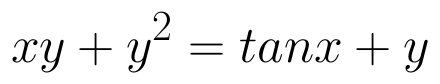. (View Answer Video)

Question 5 : If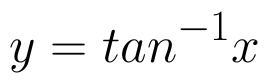, find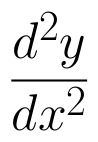in terms of y alone. (View Answer Video)

### Matrices

Question 1 : If for any square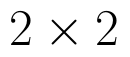matrix A,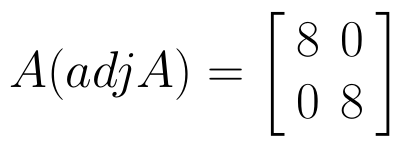then write the value of |A|. (View Answer Video)

Question 2 : If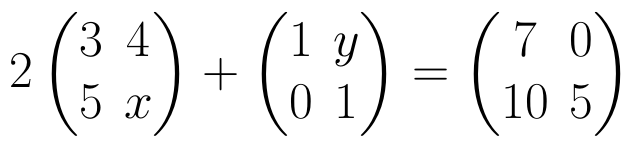, find (x-y). (View Answer Video)

Question 3 : If,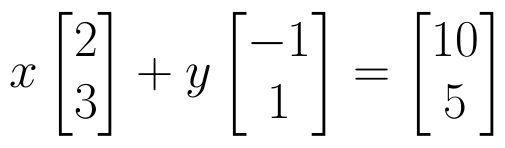find the value of y.  (View Answer Video)

Question 4 : Find the inverse of the matrix,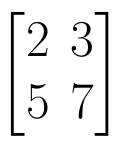. (View Answer Video)

Question 5 : Find the value of X, if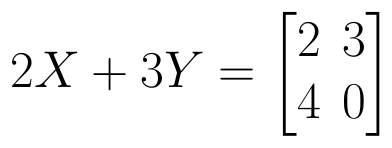and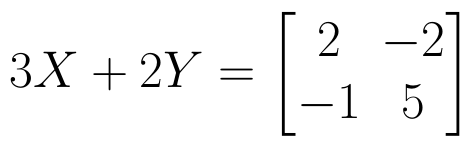. (View Answer Video)

### Relations and Functions

Question 1 :  If f(x) = ax + b and g(x) = cx + d, then f[g(x)] – g[f(x)] is equivalent to, (View Answer Video)

Question 2 :  Let R be the relation on the set {1, 2, 3, 4} given by R = {(1, 2), (2, 2), (1, 1), (4, 4), (1, 3), (3,3), (3,2)}. then R is, (View Answer Video)

Question 3 :  A function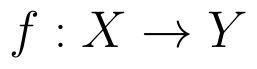is surjective if and only if , (View Answer Video)

Question 4 : Let * be the binary operation on N given by a * b = LCM of a and b. Find 20 * 16. (View Answer Video)

Question 5 : If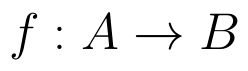and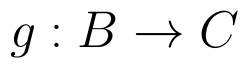are onto, then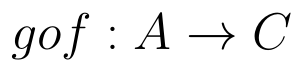is: (View Answer Video)

### Three Dimensional Geometry

Question 1 : The equation of a line AB is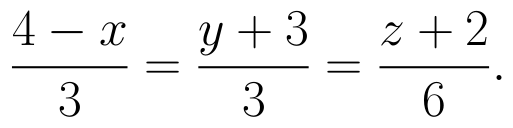Write the direction cosines of a line parallel to the above line. (View Answer Video)

Question 2 : Find the vector equation of a plane which is at a distance of 5 units from the origin and its normal vector is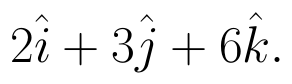(View Answer Video)

Question 3 : If a lines makes angle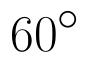and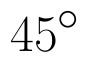with the positive directions of x-axis and z-axis respectively, then find the angle that it makes with the y-axis. (View Answer Video)

Question 4 : Find the distance of the point (-1, -5, -10) from the point of intersection of the line joining the points A(2, -1, 2) and B(5, 3, 4) with the plane x - y + z = 5. (View Answer Video)

Question 5 : Find the equation of the plane passing through the line of intersection of the planes x + y + z = 1 and 2x + 3y + 4z = 5 which is perpendicular to the plane x - y + z = 0. Also, find the distance of the plane obtained above from the origin. (View Answer Video)